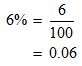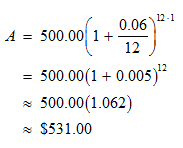# What is Monthly Compound Interest Formula?

When interest is compounded on a monthly frequency it is known as monthly compound interest. In monthly compounding interest is charged both on the principal as well as the accumulated interest. For the calculation of monthly compounding, it is important to know the principal portion the time frame and the annual interest charged by the lenders.

The equation for calculating the monthly compound interest is represented as follows,

A= (P (1+r/n)^(nt)) – P

Where

• A= Monthly compound rate

• P= Principal amount

• R= Rate of interest

• N= Time period

## Example

STEP 1: The rate of interest is 6% per year. Before you begin the calculations, you need to express 6% as an equivalent decimal number. This can be achieved by dividing 6 by 100.STEP 2: Since the interest is compounded monthly, you can take n as 12. As no time period has been specified, we shall assume that the loan is taken for a period of one year. Now that all the variable values are known, you can directly substitute them in the formula and get the result.We have determined the loan amount after 1 year by monthly compounding as about \$531.00.